## Factorio

Post

### Re: Factorio

#196
While that is removing trash, a lot of stuff still stays in my inventory.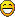Have a question? Send me a PM! || I have a Patreon page up for REKT now! || People talking in IRC over the past two hours:Post

### Re: Factorio

#197
DWMagus wrote:
Talvieno wrote:Trains can't deliver goodies and remove trash from my inventory thoughNot sure about that first one, but the second one? Ever get hit by a train?No, I'm not that stupid.I am Groot.
Please don't take my advice. You will wind up in jail if you do.
For some reason, I feel obliged to display how many people have talked in IRC over the past 2 hours: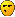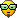Post

### Re: Factorio

#198
Baile nam Fonn wrote: With the tank-mounted flamethrower? If so, teach me the way!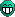Baile nam Fonn wrote:With the tank-mounted flamethrower?
Baile nam Fonn wrote:tank-mounted flamethrowerPost

### Re: Factorio

#199
0111narwhalz wrote:
Baile nam Fonn wrote: With the tank-mounted flamethrower? If so, teach me the way!Baile nam Fonn wrote:With the tank-mounted flamethrower?
Baile nam Fonn wrote:tank-mounted flamethrowerI hope you're not envisioning a mobile version of the turret. Flame jet is probably a more apt descriptor: short range, no pools of fire, continuous ankle-biter-melting output that is slightly choppy in practice since the jet has to turn off to achieve it's max radians per second (at least, it appears so to me). It has very good utility for speeding progress through forests should you wish to expedite your return trip or blaze a shortcut.
"omg such tech many efficiency WOW" ~ Josh Parnell
Post

### Re: Factorio

#200
Hmm... It's probably just using a different kind of projectile. I think turning it into the other kind would be a nice exercise.Post

### Re: Factorio

#201
The title was Specifying the 1.0, but all I heard was
FFF#189 wrote:planned gameplay features [..] artillery train"omg such tech many efficiency WOW" ~ Josh Parnell
Post

### Re: Factorio

#202
I saw that too! What could it mean? We know about the spidertron, but... artillery train? Is this like a train car that fires at enemies, long-range? If so, I can see myself making one or two dedicated artillery train cars to keep the perimeter clear.That would be fun.
Have a question? Send me a PM! || I have a Patreon page up for REKT now! || People talking in IRC over the past two hours:Post

### Re: Factorio

#203
Baile nam Fonn wrote:The title was Specifying the 1.0, but all I heard was
FFF#189 wrote:planned gameplay features [..] artillery trainWhenever I hear "artillery train" I always have the "heavy Gustav" cannon of ze germans in my mind...
Automation engineer, lateral thinker, soldier, addicted to music, books and gaming.
Nothing to see here
Flatfingers wrote: 23.01.2017: "Show me the smoldering corpse of Perfectionist Josh"
Post

### Re: Factorio

#204
Talvieno wrote:I saw that too! What could it mean? We know about the spidertron, but... artillery train? Is this like a train car that fires at enemies, long-range? If so, I can see myself making one or two dedicated artillery train cars to keep the perimeter clear.That would be fun.
with that i'd like electrical trains that i can stop bothering about refueling them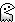Post

### Re: Factorio

#205
I don't mind refueling. It's not much more difficult than "stop at a station and get fuel handed in with inserters".
Have a question? Send me a PM! || I have a Patreon page up for REKT now! || People talking in IRC over the past two hours:Post

### Re: Factorio

#206
its the same difference between coal fueled furnaces and electrical furnaces.

Of course i have no problem fueling them, its easier to do so with the electrical ones, though.

Why shouldnt i be able to power my train system with the same power plant thats powering the rest of the factory already?
Post

### Re: Factorio

#207
I guess. I rarely rip up my original assembly line anyway. Too lazy, and I don't like shifting everything for 3x3 furnaces instead of 2x2. It's not like there's ever a shortage of coal, either.
Have a question? Send me a PM! || I have a Patreon page up for REKT now! || People talking in IRC over the past two hours:Post

### Re: Factorio

#208
Talvieno wrote:It's not like there's ever a shortage of coal, either.
Heh. For everything there is a season."omg such tech many efficiency WOW" ~ Josh Parnell
Post

### Re: Factorio

#209
Talvieno wrote:I guess. I rarely rip up my original assembly line anyway. Too lazy, and I don't like shifting everything for 3x3 furnaces instead of 2x2. It's not like there's ever a shortage of coal, either.
Use a base foundry design that is built to be expanded later into 3x3

< = Belt
L = Long Inserter
S = Short Inserter
F = Furnace
p = Power Line (basic one)

INITIAL

Code: Select all

``````<<<<<<<<<<<<<<<
p     p     p
L  L  L  L  L
FF FF FF FF FF
FF FF FF FF FF
SpLS LSpLS LSpL
<<<<<<<<<<<<<<<  Ore+Ore
<<<<<<<<<<<<<<<  Coal+Ore
<<<<<<<<<<<<<<<  Ore+Ore
SpLS LSpLS LSpL
FF FF FF FF FF
FF FF FF FF FF
L  L  L  L  L
p     p     p
<<<<<<<<<<<<<<<
``````

Code: Select all

``````<<<<<<<<<<<<<<<
Sp S  Sp S  Sp
FFFFFFFFFFFFFFF
FFFFFFFFFFFFFFF
FFFFFFFFFFFFFFF
SpLS LSpLS LSpL
<<<<<<<<<<<<<<<  Ore+Ore
<<<<<<<<<<<<<<<  Ore+Ore
<<<<<<<<<<<<<<<  Ore+Ore
SpLS LSpLS LSpL
FFFFFFFFFFFFFFF
FFFFFFFFFFFFFFF
FFFFFFFFFFFFFFF
Sp S  Sp S  Sp
<<<<<<<<<<<<<<<
``````
You only have to remove a long inserter per furnace, and upgrade each to electric.
With the three lines of Ore in, you can get a fair level of ore input and keep a LOT of furnaces going at once.
Adding another middle line instead at the start is an option, the two middle lines would then begin as ore+coal, and turn into ore+ore.
Would mean each side got four lines of ore along it's two belts.
The short inserters should be upgraded to fast ones. And you could put a line of crates in before the output lines, like below...

c = Crate

Code: Select all

``````<<<<<<<<<<<<<<<
S  S  S  S  S
c  c  c  c  c
Sp S  Sp S  Sp
FFFFFFFFFFFFFFF
FFFFFFFFFFFFFFF
FFFFFFFFFFFFFFF
SpLS LSpLS LSpL
<<<<<<<<<<<<<<<  Ore+Ore
<<<<<<<<<<<<<<<  Ore+Ore
``````
If you have exceptional output, be fancy! Have an extra output line. :V

Code: Select all

``````<<<<<<<<<<<<<<<
<<<<<<<<<<<<<<<
S  L  S  L  S
c  c  c  c  c
Sp S  Sp S  Sp
FFFFFFFFFFFFFFF
FFFFFFFFFFFFFFF
FFFFFFFFFFFFFFF
SpLS LSpLS LSpL
<<<<<<<<<<<<<<<  Ore+Ore
<<<<<<<<<<<<<<<  Ore+Ore
``````
°˖◝(ಠ‸ಠ)◜˖°
WebGL Spaceships and Trails
<Cuisinart8> apparently without the demon driving him around Silver has the intelligence of a botched lobotomy patient ~ Mar 04 2020
console.log(`What's all \${this} \${Date.now()}`);
Post

### Re: Factorio

#210
Silverware wrote:
Talvieno wrote:I guess. I rarely rip up my original assembly line anyway. Too lazy, and I don't like shifting everything for 3x3 furnaces instead of 2x2. It's not like there's ever a shortage of coal, either.
Use a base foundry design that is built to be expanded later into 3x3

< = Belt
L = Long Inserter
S = Short Inserter
F = Furnace
p = Power Line (basic one)

INITIAL

Code: Select all

``````<<<<<<<<<<<<<<<
p     p     p
L  L  L  L  L
FF FF FF FF FF
FF FF FF FF FF
SpLS LSpLS LSpL
<<<<<<<<<<<<<<<  Ore+Ore
<<<<<<<<<<<<<<<  Coal+Ore
<<<<<<<<<<<<<<<  Ore+Ore
SpLS LSpLS LSpL
FF FF FF FF FF
FF FF FF FF FF
L  L  L  L  L
p     p     p
<<<<<<<<<<<<<<<
``````

Code: Select all

``````<<<<<<<<<<<<<<<
Sp S  Sp S  Sp
FFFFFFFFFFFFFFF
FFFFFFFFFFFFFFF
FFFFFFFFFFFFFFF
SpLS LSpLS LSpL
<<<<<<<<<<<<<<<  Ore+Ore
<<<<<<<<<<<<<<<  Ore+Ore
<<<<<<<<<<<<<<<  Ore+Ore
SpLS LSpLS LSpL
FFFFFFFFFFFFFFF
FFFFFFFFFFFFFFF
FFFFFFFFFFFFFFF
Sp S  Sp S  Sp
<<<<<<<<<<<<<<<
``````
You only have to remove a long inserter per furnace, and upgrade each to electric.
With the three lines of Ore in, you can get a fair level of ore input and keep a LOT of furnaces going at once.
Adding another middle line instead at the start is an option, the two middle lines would then begin as ore+coal, and turn into ore+ore.
Would mean each side got four lines of ore along it's two belts.
The short inserters should be upgraded to fast ones. And you could put a line of crates in before the output lines, like below...

c = Crate

Code: Select all

``````<<<<<<<<<<<<<<<
S  S  S  S  S
c  c  c  c  c
Sp S  Sp S  Sp
FFFFFFFFFFFFFFF
FFFFFFFFFFFFFFF
FFFFFFFFFFFFFFF
SpLS LSpLS LSpL
<<<<<<<<<<<<<<<  Ore+Ore
<<<<<<<<<<<<<<<  Ore+Ore
``````
If you have exceptional output, be fancy! Have an extra output line. :V

Code: Select all

``````<<<<<<<<<<<<<<<
<<<<<<<<<<<<<<<
S  L  S  L  S
c  c  c  c  c
Sp S  Sp S  Sp
FFFFFFFFFFFFFFF
FFFFFFFFFFFFFFF
FFFFFFFFFFFFFFF
SpLS LSpLS LSpL
<<<<<<<<<<<<<<<  Ore+Ore
<<<<<<<<<<<<<<<  Ore+Ore
``````
I ABSOLUTELY HAVE TO PLAY FACTORIO WITH YOU!!!Automation engineer, lateral thinker, soldier, addicted to music, books and gaming.
Nothing to see here
Flatfingers wrote: 23.01.2017: "Show me the smoldering corpse of Perfectionist Josh"

### Online Now

Users browsing this forum: No registered users and 2 guests